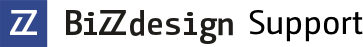Go to start of banner

# Setting a color per date range in a color view

In this topic, it is assumed you are familiar with working with a custom configuration and that you have an object with a date value.

It is possible to set a certain color per date value in a color view. It is useful if you want to highlight certain date values, for example, if you want to show threshold values using certain colors per application component. In the example below, you can see several application components with various dates. The goal of this example is to show certain values per color per date range.# How does it work?

After placing the components a color view is generated. Unfortunately, this generates random colors. In order to accomplish the goal of the example, we want to show Application components in the same color if the dates are in the same month. To achieve this, the viewpoint that has been created when generating the color view needs to be expanded with a piece of code. Edit the created viewpoint by right-clicking it in the Viewpoints pane, and then clicking Query Tool

The code below is used to create the example:

`// Start Example `
`//determine the colour, where as 0,128,0 = green `
`parameter "ValueColor" "December", List(0,128,0); `
`parameter "ValueColor" "January", List(255,0,0); `
`parameter "ValueColor" "November", List(255,0,255); `
`header translate("Startingdate")+" "+translate("Month", "en"); `
`  forall a1 in modelpackage { `
`   a2 = true; `
`    if (a1.hasAttrRef("Month")) { `
`         a3 = a1.attrValueRef("Month"); `
`       if ((a3 > Date(2015,12,1)) && (a3 < Date(2015,12,31))) { `
`       output a1, "December"; `
`      //output value: `
`      //date has to be later then 1 dec 2015 AND before 31 dec 2015 `
`      //if true, the output will be 'December'  `
`      //for this instance that will be green `
`      } `
`      if ((a3 > Date(2015,11,1)) && (a3 < Date(2015,11,30))) { `
`      output a1, "November"; `
`      //date has to be later then 1 nov 2015 AND before 30 nov 2015 `
`      //if true, the output will be 'November' `
`      //for this instance that will be purple `
`      } `
`      if ((a3 > Date(2016,1,1)) && (a3 < Date(2016,1,31))) { `
`      output a1, "January"; `
`      //date has to be later then 1 jan 2016 AND before 31 jan 2016 `
`      //if true, the output will be 'January' `
`      //for this instance that will be red `
`      }`
`   }`
`} `
`//End Example`

Note that Application components 5 and 9 in the above example figure are blank since their date does not start in November, December or January.

Below you can find the example model:

Examplemodel_colorscript.zip

• Page:
• Page:
• Page:
• Page:
• Page:
• Page:

• Page: